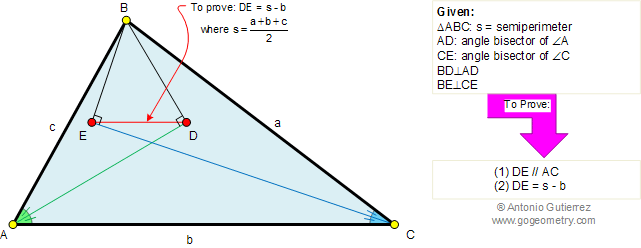Problem 385. Triangle, Angle bisector, Perpendicular, Parallel, Semiperimeter. Level: High School, SAT Prep, College geometry. The figure shows a triangle ABC with angle bisectors AD and CE of angles A and C, respectively. BD is perpendicular to AD and BE is perpendicular to CE. Prove that (1) DE is parallel to AC. (2) If s is the semiperimeter of triangle ABC, DE = s - b.Recent Additions